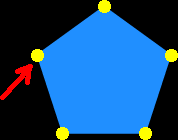# Online Math Dictionary: V

Easy to understand math definitions for K-Algebra mathematics
Just scroll down or click on the word you want and I'll scroll down for you!

 variable vector vertex vertical volume

Variable
In Algebra, a variable is the letter in an equation that represents an unknown number.
Examples:  2x + 5 = 7       2x - 3y = 6VectorA vector is a ray that has a magnitude (length) and a direction (which way it's pointing.)  Vectors are used a lot in physics and navigation.VertexOn a polygon, the vertices (the plural of vertex) are the corners.In a parabola, the vertex is the starting point.  The line that cuts the parabolain half (the axis of symmetry) goes right through the vertex.VerticalA vertical line is a line that goes up and down...  A vertical line isperpendicular to the horizon.